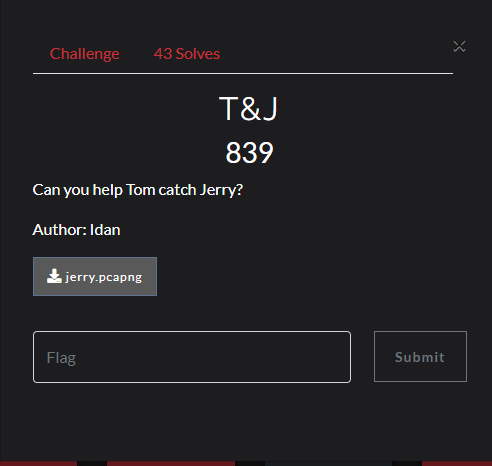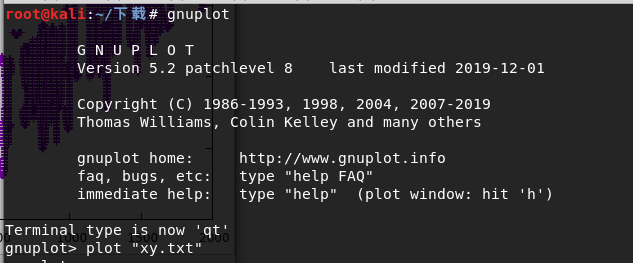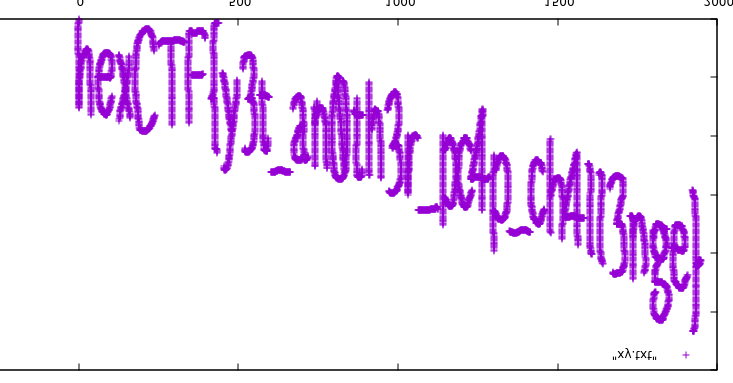## [HexionCTF] T&J## 题目详解

``tshark -r jerry.pcapng -T fields -e usb.capdata | sed '/^\s*\$/d' > usbdata.txt``

``````f=open('usbdata.txt','r')
fi=open('out.txt','w')
while 1:
if a:
if len(a)==16: # 鼠标流量的话len改为8
out=''
for i in range(0,len(a),2):
if i+2 != len(a):
out+=a[i]+a[i+1]+":"
else:
out+=a[i]+a[i+1]
fi.write(out)
fi.write('\n')
else:
break

fi.close()``````

``````nums = []
keys = open('out.txt','r')
f = open('xy.txt','w')
posx = 0
posy = 0
for line in keys:
#    if len(line) != 12 :
#        continue
x = int(line[3:5],16)
y = int(line[6:8],16)
if x > 127 :
x -= 256
if y > 127 :
y -= 256
posx += x
posy += y
btn_flag = int(line[0:2],16)  # 1 for left , 2 for right , 0 for nothing
if btn_flag == 1 : # 1 代表左键
f.write(str(posx))
f.write(' ')
f.write(str(posy))
f.write('\n')

f.close()``````• 注意plot后一定要用双引号包含文件的名称

``convert -flip 1.png 2.png``